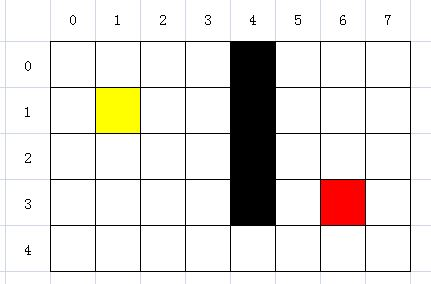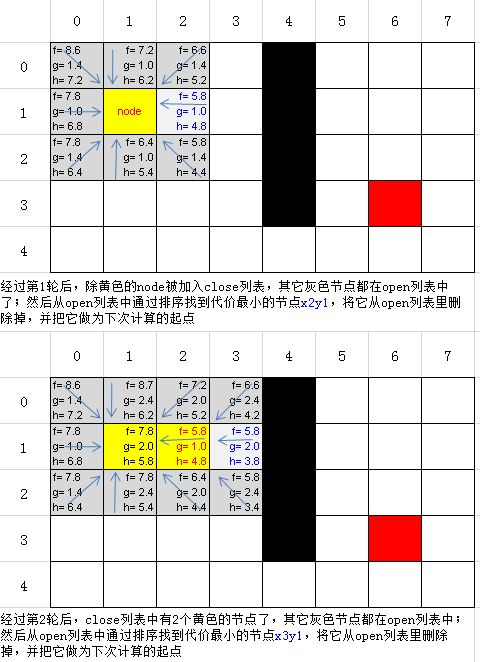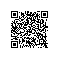# “AS3.0高级动画编程”学习：第四章 寻路（AStar/A星/A*）算法 (中)1、先以起点为中心，向周边扩张一圈，同时计算出周边节点(最多有8个)的单步代价g(即从中心点移动到相邻格子的代价：水平或垂直为1，对角为1.4)；然后再计算周边每个节点到终点的估算代价h(利用上一部分最后讲到的估算公式)，从而得出周围每个节点的总代价 f = g+h

2、同时准备二个数组，一个称为开放列表(open)，一个称为封闭列表(closed)，把周边节点放入open数组当中，然后利用数组排序对周边节点的f值从小到大排序，从而找出最小代价的节点----代价最小，意味着应该向这个节点移动。然后再把本轮计算中的中心点放入close数组(也就是意味着该既然找到了下一步，这一步就不用再考虑了)

3、把第2步中得到的代价最小的节点做为中心点，同时从open数组中删除该节点（因为既然已经找到了正确的一步，这一个节点就不用参与下次的排序了），继续向外扩展一圈，得到新的周边节点，同样计算g值和h值，但有一点要注意：因为现在的中心不是刚才的起点了，所以g值的计算实际由二部分组成，一部分为本次中心节点距离起点的g值(记为g1)，一部分为本次中心节点周围节点的g值(记为g2)，最终每个周边节点的g = g1 + g2；而且还有一个要注意的地方：节点的相对位置可能发生变化，比如在前一轮中某些节点相对于前一轮中心节点处于水平或垂直位置，而在本轮计算中，由于中心点向前移动了一位，所以同样的节点在本轮计算时，可能会变成对中心节点的对角节点（即g2由1变成1.4），反之亦然；h值计算跟以前一样，类似前面的处理，得到最终代价f = g + h，同样：把新的周边节点放入open数组(如果还没有放入的话)，对于在第2步中就已经放入open数组或close数组的节点，因为g值有可能发生变化了，所以也要重新比较本次得到的总代价f，跟上次计算得到的总代价f，如果本次计算的f值更小，则以本次计算的f值为准(把上一轮节点的f,h,g值更新掉)，然后同样的处理，对open数组进行f值从小到大排序，得到代价最小的新节点，做为下一轮计算的中心。(当然下一轮计算前，同样要把已经找到的代价最小的节点从open数组中删除，同时把本次计算的中心点放入close数组)

4、按照前面的处理，如此这般反复下去，路径会一直不断的延伸下去（当然延伸的过程可能会有曲折或反复），但因为我们一直是在取代价最小的节点，所以总体来讲，肯定是越来越靠近终点。

5、在上面反复处理的过程中，一旦发现本轮计算的中心点就是终点，那么恭喜你，我们走到终点了！

package
{
import flash.display.Sprite;

public class GridTest extends Sprite
{
private var _endNode:Node;//终点
private var _startNode:Node;//起点
private var _centerNode:Node;//本次计算的中心点
private var _straightCost:Number=1.0;
private var _diagCost:Number=Math.SQRT2;

public function GridTest()
{
var g:Grid=new Grid(8, 5);//生成一个5行8列的网格
g.setStartNode(1, 1);//设置起点
g.setEndNode(6, 3); //设置终点

_endNode=g.endNode;
_startNode=g.startNode;
_centerNode = g.getNode(2,1);//大家可以调整此中心点位置，以观察周边节点的f,h,g值

//这里借用了ascb第三方库，对数字进行格式化，取小数点后一个小数（如果大家没有ascb官方库，也可以直接输出数字）
var fmr:NumberFormat = new NumberFormat();

var _g1:Number = diagonal(_centerNode,_startNode);//中心点相对起点的g值

//这里的x即为中心点周围节点的x范围(可手动调整)
for (var x:uint=1; x <= 3; x++)
{
//这里的y即为中心点周围节点的y范围(可手动调整)
for (var y:uint=0; y <= 2; y++)
{
var test:Node=g.getNode(x, y);
var _h:Number=diagonal(test,_endNode);
var _g2:Number = diagonal(test,_centerNode);
var _g:Number=_g1 + _g2;//计算g值
var _f:Number=_h+_g;
trace("x=", test.x, "，y=", test.y, "，f=", fmr.format(_f), "，g=", fmr.format(_g), "，h=", fmr.format(_h));
}
}
}

//对角线估价法
private function diagonal(node:Node,target:Node):Number
{
var dx:Number=Math.abs(node.x - target.x);
var dy:Number=Math.abs(node.y - target.y);
var diag:Number=Math.min(dx, dy);
var straight:Number=dx + dy;
return _diagCost * diag + _straightCost * (straight - 2 * diag);
}
}
}


x= 1 ，y= 0 ，f= 8.7 ，g= 2.4 ，h= 6.2
x= 1 ，y= 1 ，f= 7.8 ，g= 2.0 ，h= 5.8
x= 1 ，y= 2 ，f= 7.8 ，g= 2.4 ，h= 5.4
x= 2 ，y= 0 ，f= 7.2 ，g= 2.0 ，h= 5.2
x= 2 ，y= 1 ，f= 5.8 ，g= 1.0 ，h= 4.8
x= 2 ，y= 2 ，f= 6.4 ，g= 2.0 ，h= 4.4
x= 3 ，y= 0 ，f= 6.7 ，g= 2.4 ，h= 4.2
x= 3 ，y= 1 ，f= 5.8 ，g= 2.0 ，h= 3.8
x= 3 ，y= 2 ，f= 5.8 ，g= 2.4 ，h= 3.4

ok，还有一个重要问题，按上面的处理，我们就算走到了终点，也得到最后一个中心点(其实就是终点)，如何把路径给构建(还原)出来呢？因为我们在处理过程中，并没有其它变量用来保存中间计算的结果(即每次找出的最小代价结节)？另外：就算用一个变量做为中介来保存每轮计算中的最佳节点，前面也提到了，向周边探讨寻路的过程中，完全有可能出现曲折反复的过程，难道最终找到的路径还要往回绕个圈（或打个结）走吗？如果是这样，那就违背了我们上一部分里寻找最佳(最短)路径的初衷。package
{
import flash.display.Sprite;

public class AStar extends Sprite
{
private var _open:Array;//开放列表
private var _closed:Array;//封闭列表
private var _grid:Grid;
private var _endNode:Node;//终点
private var _startNode:Node;//起点
private var _path:Array;//最终的路径节点
// private var _heuristic:Function = manhattan;
// private var _heuristic:Function = euclidian;
private var _heuristic:Function=diagonal; //估计公式
private var _straightCost:Number=1.0; //直线代价
private var _diagCost:Number=Math.SQRT2; //对角线代价

public function AStar()
{

}

//判断节点是否开放列表
private function isOpen(node:Node):Boolean
{
for (var i:int=0; i < _open.length; i++)
{
if (_open[i] == node)
{
return true;
}
}
return false;
}

//判断节点是否封闭列表
private function isClosed(node:Node):Boolean
{
for (var i:int=0; i < _closed.length; i++)
{
if (_closed[i] == node)
{
return true;
}
}
return false;
}

//对指定的网络寻找路径
public function findPath(grid:Grid):Boolean
{
_grid=grid;
_open=new Array();
_closed=new Array();
_startNode=_grid.startNode;
_endNode=_grid.endNode;
_startNode.g=0;
_startNode.h=_heuristic(_startNode);
_startNode.f=_startNode.g + _startNode.h;
return search();
}

//计算周围节点代价的关键处理函数
public function search():Boolean
{
var _t:uint =1;
var node:Node=_startNode;
//如果当前节点不是终点
while (node != _endNode)
{
//找出相邻节点的x,y范围
var startX:int=Math.max(0, node.x - 1);
var endX:int=Math.min(_grid.numCols - 1, node.x + 1);
var startY:int=Math.max(0, node.y - 1);
var endY:int=Math.min(_grid.numRows - 1, node.y + 1);

//循环处理所有相邻节点
for (var i:int=startX; i <= endX; i++)
{
for (var j:int=startY; j <= endY; j++)
{
var test:Node=_grid.getNode(i, j);
//如果是当前节点，或者是不可通过的，则跳过
if (test == node || !test.walkable)
{
continue;
}

var cost:Number=_straightCost;
//如果是对象线，则使用对角代价
if (!((node.x == test.x) || (node.y == test.y)))
{
cost=_diagCost;
}

//计算test节点的总代价
var g:Number=node.g + cost * test.costMultiplier;
var h:Number=_heuristic(test);
var f:Number=g + h;

//如果该点在open或close列表中
if (isOpen(test) || isClosed(test))
{
//如果本次计算的代价更小，则以本次计算为准
if (f<test.f)
{
trace("\n第",_t,"轮，有节点重新指向，x=",i,"，y=",j,"，g=",g,"，h=",h,"，f=",f,"，test=",test.toString());
test.f=f;
test.g=g;
test.h=h;
test.parent=node;//重新指定该点的父节点为本轮计算中心点
}
}
else//如果还不在open列表中，则除了更新代价以及设置父节点，还要加入open数组
{
test.f=f;
test.g=g;
test.h=h;
test.parent=node;
_open.push(test);
}
}
}
_closed.push(node);//把处理过的本轮中心节点加入close节点

//辅助调试，输出open数组中都有哪些节点
for(i=0;i<_open.length;i++){
trace(_open[i].toString());
}

if (_open.length == 0)
{
trace("没找到最佳节点，无路可走!");
return false
}
_open.sortOn("f", Array.NUMERIC);//按总代价从小到大排序
node=_open.shift() as Node;//从open数组中删除代价最小的结节，同时把该节点赋值为node，做为下次的中心点
trace("第",_t,"轮取出的最佳节点为：",node.toString());
_t++;
}
//循环结束后，构建路径
buildPath();
return true;
}

//根据父节点指向，从终点反向连接到起点
private function buildPath():void
{
_path=new Array();
var node:Node=_endNode;
_path.push(node);
while (node != _startNode)
{
node=node.parent;
_path.unshift(node);
}
}

//曼哈顿估价法
private function manhattan(node:Node):Number
{
return Math.abs(node.x - _endNode.x) * _straightCost + Math.abs(node.y - _endNode.y) * _straightCost;
}

//几何估价法
private function euclidian(node:Node):Number
{
var dx:Number=node.x - _endNode.x;
var dy:Number=node.y - _endNode.y;
return Math.sqrt(dx * dx + dy * dy) * _straightCost;
}

//对角线估价法
private function diagonal(node:Node):Number
{
var dx:Number=Math.abs(node.x - _endNode.x);
var dy:Number=Math.abs(node.y - _endNode.y);
var diag:Number=Math.min(dx, dy);
var straight:Number=dx + dy;
return _diagCost * diag + _straightCost * (straight - 2 * diag);
}

//返回所有被计算过的节点(辅助函数)
public function get visited():Array
{
return _closed.concat(_open);
}

//返回open数组
public function get openArray():Array{
return this._open;
}

//返回close数组
public function get closedArray():Array{
return this._closed;
}

public function get path():Array
{
return _path;
}
}
}


		//方便调试输出用的toString函数
public function toString():String{
var fmr:NumberFormat = new NumberFormat();
return "x=" + this.x.toString() + ",y=" + this.y.toString() + ",g=" + fmr.format(this.g) + ",h=" + fmr.format(this.h) + ",f=" + fmr.format(this.f);
}


package
{
import flash.display.Sprite;
import flash.events.MouseEvent;

public class GridView extends Sprite
{
private var _cellSize:int=40;
private var _grid:Grid;

//构造函数
public function GridView(grid:Grid)
{
_grid=grid;
drawGrid();
findPath();
}

//画格子
public function drawGrid():void
{
graphics.clear();
for (var i:int=0; i < _grid.numCols; i++)
{
for (var j:int=0; j < _grid.numRows; j++)
{
var node:Node=_grid.getNode(i, j);
graphics.lineStyle(0);
graphics.beginFill(getColor(node));
graphics.drawRect(i * _cellSize, j * _cellSize, _cellSize, _cellSize);
}
}
}

//取得节点颜色
private function getColor(node:Node):uint
{
if (!node.walkable)
{
return 0;
}
if (node == _grid.startNode)
{
return 0xffff00;
}
if (node == _grid.endNode)
{
return 0xff0000;
}
return 0xffffff;
}

//网格点击事件
private function onGridClick(event:MouseEvent):void
{
var xpos:int=Math.floor(event.localX / _cellSize);
var ypos:int=Math.floor(event.localY / _cellSize);
_grid.setWalkable(xpos, ypos, !_grid.getNode(xpos, ypos).walkable);
drawGrid();
findPath();
}

//寻找路径
private function findPath():void
{
var astar:AStar=new AStar;
if (astar.findPath(_grid))
{
showVisited(astar);
showPath(astar);
}
}

//显示open列表与close列表
private function showVisited(astar:AStar):void
{

var opened:Array=astar.openArray;
for (var i:int=0; i < opened.length; i++)
{
var node:Node = opened[i] as Node;

graphics.beginFill(0xcccccc);
if (node==_grid.startNode){
graphics.beginFill(0xffff00);
}

graphics.drawRect(opened[i].x * _cellSize, opened[i].y * _cellSize, _cellSize, _cellSize);
graphics.endFill();
}

var closed:Array=astar.closedArray;
for (i=0; i < closed.length; i++)
{
node = opened[i] as Node;

graphics.beginFill(0xffff00);

graphics.drawRect(closed[i].x * _cellSize, closed[i].y * _cellSize, _cellSize, _cellSize);
graphics.endFill();
}
}

//显示路径
private function showPath(astar:AStar):void
{
var path:Array=astar.path;
for (var i:int=0; i < path.length; i++)
{
graphics.lineStyle(0);
graphics.beginFill(0);
graphics.drawCircle(path[i].x * _cellSize + _cellSize / 2, path[i].y * _cellSize + _cellSize / 2, _cellSize / 3);
}
}
}
}


package
{
import flash.display.Sprite;
import flash.display.StageAlign;
import flash.display.StageScaleMode;
import flash.events.MouseEvent;

[SWF(backgroundColor=0xffffff,width=360,height=240)]
public class Pathfinding extends Sprite
{
private var _grid:Grid;
private var _gridView:GridView;

public function Pathfinding()
{
stage.align=StageAlign.TOP_LEFT;
stage.scaleMode=StageScaleMode.NO_SCALE;
_grid=new Grid(8, 5);
_grid.setStartNode(1, 1);
_grid.setEndNode(6, 3);

//设置障碍物
_grid.getNode(4,0).walkable = false;
_grid.getNode(4,1).walkable = false;
_grid.getNode(4,2).walkable = false;
_grid.getNode(4,3).walkable = false;

_gridView=new GridView(_grid);
_gridView.x=20;
_gridView.y=20;
}
}
}使用钉钉扫一扫加入圈子
+ 订阅